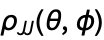# Visualizing Polarization Moments and Quantum Beats

Here we graphically illustrate the angular-momentum polarization of static and dynamic atomic systems using functions from the AtomicDensityMatrix package.

 Set graphics options.

Here is a density matrix representing an aligned spin-1 system.

We can see that the density matrix contains only population andalignment by decomposing it into its irreducible tensor components using Decomposition. A list containing one rank-0 (one component), one rank-1 (three components), and one rank-2 (five components) irreducible tensor is produced. Irreducible tensors are indicated by double sets of curly brackets.

 Display the decomposition of the density matrix as a list of column vectors using TensorForm.

The angular-momentum probability surface is the plot of ρJJ(θ,ϕ), where θ and ϕ represent the direction of the quantization axis. This is the matrix element JJ(θ,ϕ)|ρ|JJ(θ,ϕ), where |JM(θ,ϕ) are the angular-momentum eigenstates with quantization axis (θ,ϕ). The surface shows the probability of measuring the maximum projection of angular momentum in the (θ,ϕ) direction.

 The function MaxProjectionProbability generates the formula for the probability surface.
 MaxProjectionProbability can also be applied to the tensor decomposition. Then ρJJ(θ,ϕ) is found as the sum of spherical harmonics.
 These two methods are equivalent.

To plot the probability surface, we can use SphericalPlot3D. Evaluate is used so that the expression foris found prior to plotting, instead of for each plot point. Axes3D adds the axes and labels.

 The angular-momentum probability surface.
 The AMPSPlot function is a shorthand to produce an angular-momentum probability surface plot directly from the density matrix or tensor decomposition.

WignerRotate can be used on either the density matrix or the tensor decomposition to perform rotations by Euler angles α,β,γ.

 Here we rotate by -π/4 about the y axis, and then π/4 about the z axis.
 And the plot.